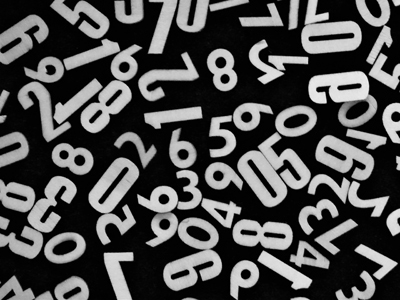There are an infinite amount of numbers - and they all have certain properties.

# Properties of Numbers 2 (Medium)

This is the second of our Medium level Eleven Plus maths quizzes on Properties of Numbers. There are several different properties, such as multiples, squares, primes, odds and evens. Do you know what they are?

If the answer is ‘No’, then please have a go at our first quiz on the topic. If you’ve already played it, then this quiz will give you more opportunity to practice, cementing the terms you have learned in your mind.

So, if you are ready, it’s time to begin. Make sure that you read each question carefully before you choose your answer. You’d be surprised how many exams are failed because students did not fully understand the question. It’s good practice to always double check your answers before you click on them.

Good luck!

1.
If a number is divisible by 6, what must the final digit of the number be?
4 or 8
0, 2, 4, 6, or 8
2, 4 or 8
0, 1, 2, 4, 6, or 8
Knowing your 6 times table will give you the answer to this one
2.
Which of the following statements is true?
There are more positive numbers than negative numbers
For every positive number there is a negative number
The smallest negative even number is -2
The smallest negative odd number is -1
For every positive number there is a negative number. -1 is the HIGHEST negative odd number and -2 is the HIGHEST negative even number – negative numbers get lower and lower as you count backwards
3.
How can an even number always be made into an odd number?
Add an odd number to it
Multiply it by 2
Add an even number to it
Divide it by 2
Adding an even number or multiplying by 2 will result in an even number. Dividing by 2 could produce an odd number, an even number or a fraction. Adding an odd number to an even number always results in an odd number
4.
Which words do not describe the numbers 5, 10, 15 and 20?
Multiples of 5
Whole numbers
Each number is 5 more than the previous number
Even numbers
They are all whole numbers, and multiples of 5. Also, each number is five more than the previous number but 5 and 15 are not even
5.
Which words do not describe the numbers 3, 6, 9 and 12?
Factors of 36
Whole numbers
Square numbers
Multiples of three
They are all whole numbers, factors of 36 and multiples of 3. The only square number in the group is 9
6.
Which of the following is not a square number?
4
9
12
16
A square number is formed by multiplying another number with itself : 4 = 2 × 2; 9 = 3 × 3; 16 = 4 × 4. Note: 1 × 1 = 1
7.
If a number is divisible by 4, what must the final digit of the number be?
4 or 8
0, 2, 4, 6, or 8
2, 4 or 8
0, 1, 2, 4, 6, or 8
Knowing your 4 times table will give you the answer to this one
8.
Which of the following statements is wrong?
If a number is divisible by 3, it is divisible by 6
If a number is a multiple of 10, it is divisible by both 2 and 5
If a number is divisible by 6, it is an even number
If a number is divisible by 6, it is divisible by 2
Not so, e.g. 3 × 3 = 9 BUT 9 is NOT divisible by 6. If you want to show that a statement is not always true, find one example that shows it is wrong - like we did here
9.
What do you always get when you multiply a positive whole number and a negative whole number?
Either a positive or negative number
Either a positive number or negative number or zero
A positive number
A negative number
For example: 2 x -4 = -8 (a negative number); 9 x -7 = -63 (a negative number); 4 x -5 = -200 (a negative number)
10.
What is a multiple of a number?
A number that is divisible exactly by the first number
An odd number
The number you get when you multiply the first number by itself
An even number
If a number is a multiple of another number, then it is divisible by the other number exactly a certain number of times, e.g. 3 goes into 18 exactly 6 times because 3 × 6 = 18
Author:  Frank Evans